Get inspired by the success stories of our students in IIT JAM MS, ISI  MStat, CMI MSc DS.  Learn More

# Mean Square Error | ISI MStat 2019 PSB Problem 5This problem based on the calculation of Mean Square Error gives a detailed solution to ISI M.Stat 2019 PSB Problem 5, with a tinge of simulation and code.

## Problem

Suppose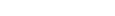are independent random variables such that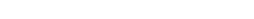where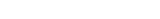are all distinct and unknown. Consider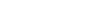and another random variable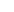which is distributed as Binomial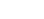where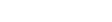Between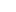and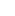which is a better estimator of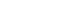in terms of their respective mean squared errors?

## Solution

#### Unbiasedness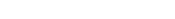.~ Binomial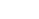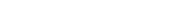.

#### Mean Square Error

If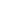is unbiased for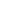, then MSE(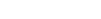.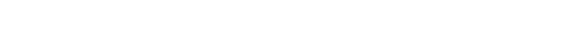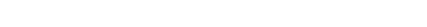Observe that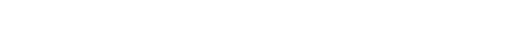.

This results in the fact that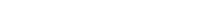.

Therefore,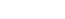is a better estimate thatnw.r.t Mean Square Error.

Let's verify this as usual by simulation.

## Computation and Simulation

library(statip)
library(Metrics)
N = 10
p = runif(10, 0, 1)
X = rep(0,N)
vX = NULL
vY = NULL
for (j in 1:1000)
{
for (i in 1:N)
{
X[i] = rbern(1,p[i])
}
Z = sum(X) #sum of Xi random variables
Y = rbinom(1,N,mean(p)) #Y random variable
vX = c(vX, Z)
vY = c(vY, Y)

}
k = rep(sum(p), 1000)
mse(k, vX) #MSE of Sum Xi #1.57966
mse(k, vY) #MSE of Y  #2.272519

Hence, the theory is verified by this simulation. I hope it helps.

This problem based on the calculation of Mean Square Error gives a detailed solution to ISI M.Stat 2019 PSB Problem 5, with a tinge of simulation and code.

## Problem

Supposeare independent random variables such thatwhereare all distinct and unknown. Considerand another random variablewhich is distributed as BinomialwhereBetweenandwhich is a better estimator ofin terms of their respective mean squared errors?

## Solution

#### Unbiasedness.~ Binomial.

#### Mean Square Error

Ifis unbiased for, then MSE(.Observe that.

This results in the fact that.

Therefore,is a better estimate thatnw.r.t Mean Square Error.

Let's verify this as usual by simulation.

## Computation and Simulation

library(statip)
library(Metrics)
N = 10
p = runif(10, 0, 1)
X = rep(0,N)
vX = NULL
vY = NULL
for (j in 1:1000)
{
for (i in 1:N)
{
X[i] = rbern(1,p[i])
}
Z = sum(X) #sum of Xi random variables
Y = rbinom(1,N,mean(p)) #Y random variable
vX = c(vX, Z)
vY = c(vY, Y)

}
k = rep(sum(p), 1000)
mse(k, vX) #MSE of Sum Xi #1.57966
mse(k, vY) #MSE of Y  #2.272519

Hence, the theory is verified by this simulation. I hope it helps.

This site uses Akismet to reduce spam. Learn how your comment data is processed.

### Knowledge Partner